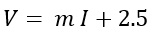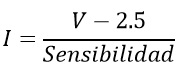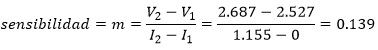ACS712 current sensor tutorialNov 29th, 2019 | by: Rick Sanchez | Views 4173
Views 4173

The ACS712 current sensor is an economical solution to measure current, internally it works with a Hall effect sensor that detects the magnetic field that is produced by induction of the current flowing through the line being measured. The sensor delivers a voltage output proportional to the current, depending on the application we can use the ACS712-05A, ACS712-20A or the ACS712-30A, for ranges of 5, 20 or 30 amps respectively

The ACS712 can be found in modules, which facilitate their connection, bring a terminal block to connect the line we want to measure and 3 pins, two to connect the power and a pin for the analog output.

The ACS712 current sensor

The ACS712 can be found in modules, which facilitate their connection, bring a terminal block to connect the line we want to measure and 3 pins, two to connect the power and a pin for the analog output.

The current range that we can measure and sensitivity vary depending on the integrated model, there are three models which we detail below:

Model

Rank

Sensitivity

ACS712ELCTR-05B-T

-5 to 5 A

185 mV / A

ACS712ELCTR-20A-T

-20 to 20 A

100 mV / A

ACS712ELCTR-30A-T

-30 to 30 A

66 mV / A

The sensor gives us a value of 2.5 volts for a current of 0A and from there increases proportionally according to the sensitivity, having a linear relationship between the voltage output of the sensor and the current.
This relationship is a straight line on a Voltage vs. Current graph where the slope is the sensitivity and the intersection on the Y axis is 2.5 volts. The equation of the line would be as followsWhere the slope is m equals Sensitivity

Clearing we will have the equation to find the current from the sensor reading:With this equation we can proceed to make the examples with Arduino.Connections between Arduino and ACS712 module

For the connections in the module guided by the names of the pins, in some models they come in different order, in the terminal block enter the line from which you want to measure, to measure the current you must connect in series with the device or load, Never connect in parallel to the voltage source.Ex.1 Making current measurements with the ACS712

To perform the current reading, you simply need to read the analog input and with the formula described above, obtain the current. Below is the code for a current reading: In our case we are working with a 5A sensor so we use the sensitivity value of 0.185V / A which is the equivalent 185mV / A that the manufacturer gives us, if they are working with the 20A sensor, replace the sensitivity value by 0.100 V / A. Below we show the results obtained from the serial monitor:

``````float  Sensitivity = 0.185 ;  // sensitivity in Volts / Amp for 5A sensor

void  setup ( )  {
Serial . begin ( 9600 ) ;
}

void  loop ( )  {
float  voltage Sensor =  analogRead ( A0 ) * ( 5.0  /  1023.0 ) ;  //
float  sensor reading    I = ( Sensor voltage - 2.5 ) / Sensitivity ;  // Equation to obtain the
Serial current . print ( "Current:" ) ;
Serial . println ( I , 3 ) ;
delay ( 200 ) ;
}
``````Ex.2 Applying Filter to ACS712 readings

The previous image is for a reading of a current of 0A, note that there is noise in the reading, if for your application the present noise does not have much importance, they could work with the program of this exercise, but a filter will have to be applied, shown below.  There are several types of filters, which depending on the complexity can consume resources in the programming of our Arduino, in our case we will simply use the arithmetic mean of several consecutive readings, implementing the average of the readings in Arduino is simple and easy to understand, simply the readings must be added and divided into the number of samples. The number of samples to calculate the average depends on the level of noise they have.

The program would be as follows below. In our case, because there is a lot of noise we work with 200 samples, the greater the quantity of samples we will obtain a better result but also the longer the time it takes for the Arduino to take the measurement, for 200 Arduino samples it takes around 35 milliseconds that can be considered negligible in most applications. Note that now the noise is of 10mA amplitude compared to the previous example that was greater than 100mA, Do not confuse the noise with the offset error that in this case is approximately +150 mA, this last error if it is representative we can subtract the result in the current equation. The following result is for a load of approximately 1.2A

``````float Sensibilidad=0.185; //sensibilidad en Voltios/Amperio para sensor de 5A

void setup() {
Serial.begin(9600);
}

void loop() {
float I=get_corriente(200);//obtenemos la corriente promedio de 500 muestras
Serial.print("Corriente: ");
Serial.println(I,3);
delay(100);
}

float get_corriente(int n_muestras)
{
float voltajeSensor;
float corriente=0;
for(int i=0;i<n_muestras;i++)
{
voltajeSensor = analogRead(A0) * (5.0 / 1023.0);////lectura del sensor
corriente=corriente+(voltajeSensor-2.5)/Sensibilidad; //Ecuación  para obtener la corriente
}
corriente=corriente/n_muestras;
return(corriente);
}
``````

Ex. 3 Calibrating our ACS712

From the sensor we obtain a measurement of approximately 1.02A, but if we measure with a multitester or ammeter the value of the current is 1.15, in our case this gives us an error of -130 mA that is different from the offset or the error for a current of 0A. If your results are also like ours, it means that the equation we are using to calculate the current is not adequate, to correct this it is necessary to calibrate our sensor and find the new sensitivity values ​​and the voltage equivalent to 0A, values ​​that We need for the equation. f the error we get with the previous examples is large, we must recalibrate and find the real values ​​since the values ​​that the manufacturer gives us are not exact. Remember the equation we use to find the current:In this equation we only have two constants: the 2.5 which is the voltage of the sensor when the current is 0V and the sensitivity, which is equivalent to the slope of the line Voltage Vs Current; we have to calculate the real values ​​of these two constants. Being a line, just get two points and with these we can calculate constants. For this we need a multitester or ammeter, which must be of good precision, since this will be the tool to calibrate. The ammeter, the ACS712 and the load must be connected in series. After connecting the ammeter and the sensor, we must take sensor voltage readings, this is done with the following program.

``````void setup() {
Serial.begin(9600);
}

void loop() {
float voltajeSensor =get_voltage(10000);//obtenemos voltaje del sensor(10000 muestras)
Serial.print("Voltaje del sensor: ");
Serial.println(voltajeSensor ,3);
}

float get_voltage(int n_muestras)
{
float voltage=0;
for(int i=0;i<n_muestras;i++)
{
voltage =voltage+analogRead(A0) * (5.0 / 1023.0);
}
voltage=voltage/n_muestras;
return(voltage);
}
``````Still calibrating our ACS712

This is one of the constants, in our case 2,527, which corresponds to point P1 (2,527.0) To find the second constant we need a second measurement, it is recommended that it is not close to the value of the first measurement, in our case we will use a load whose current is greater than 1 amp. As you can see the sensor has a voltage of 2,687, and in the ammeter we measure 1,155A, this would be our second point P2 (2,687,1555), to calculate the sensitivity we simply calculate the slope. Having these two values ​​the equation to calculate the current is:Where sensitivity = 0.139 V / A

With these new values ​​we must work the previous examples

Keep in mind that each sensor has its own characteristic

Another way can be to take several points and finally apply regression and find the equation of the line.Ex. 4 Watt and AC Ammeter using the ACS712 module

n this example we will make measurements of alternating current intensity and with this calculate the power. When we perform a reading of the ACS712 we obtain the current value at that moment, said value will be oscillating at a frequency of 60Hz, the maximum values ​​at which it oscillates are the peak currents, our program must then be able to obtain the peak values ​​of the wave of current, Having the value of the Peak current we can calculate the effective current or RMS and with this the power. Below we show the code of this example below. The algorithm consists of making measurements for half a second, which is equivalent to making measurements for 30 cycles (60Hz signal), during this time we obtain the maximum and minimum readings that correspond to + Ip and –Ip, the current Ip will be the average of these. To this result we subtract the amplitude of the noise that is present when the current is 0. Note that to reduce the noise a bit we apply a low pass filter, which is similar to an average of 10 samples.

current = 0.9 * current + 0.1 * ( ( voltajeSensor - 2527 ) / Sensitivity ) ; // Equation to get the current

With these weights we muffle the noise a bit without affecting the 60Hz signal. Below is the result when we have no load connected (0 amps)

``````float Sensibilidad=0.139; //sensibilidad en V/A para nuestro sensor
float offset=0.100; // Equivale a la amplitud del ruido
void setup() {
Serial.begin(9600);
}

void loop() {
float Ip=get_corriente();//obtenemos la corriente pico
float Irms=Ip*0.707; //Intensidad RMS = Ipico/(2^1/2)
float P=Irms*220.0; // P=IV watts
Serial.print("Ip: ");
Serial.print(Ip,3);
Serial.print("A , Irms: ");
Serial.print(Irms,3);
Serial.print("A, Potencia: ");
Serial.print(P,3);
Serial.println("W");
delay(500);
}

float get_corriente()
{
float voltajeSensor;
float corriente=0;
long tiempo=millis();
float Imax=0;
float Imin=0;
while(millis()-tiempo<500)//realizamos mediciones durante 0.5 segundos
{
voltajeSensor = analogRead(A0) * (5.0 / 1023.0);//lectura del sensor
corriente=0.9*corriente+0.1*((voltajeSensor-2.527)/Sensibilidad); //Ecuación  para obtener la corriente
if(corriente>Imax)Imax=corriente;
if(corriente<Imin)Imin=corriente;
}
return(((Imax-Imin)/2)-offset);
}
``````